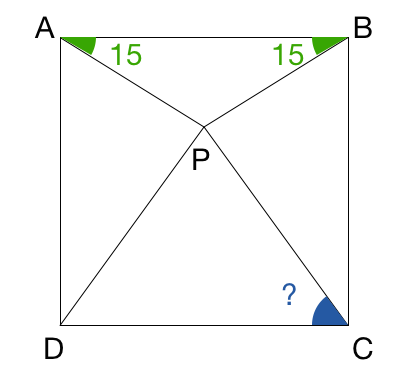# Is Trigonometry Mandatory?

Geometry Level 1Let there exist a point $P$ inside square $ABCD$ such that $\angle PAB = \angle PBA = 15^{\circ}$. Find $\angle PCD$ in degrees.

×